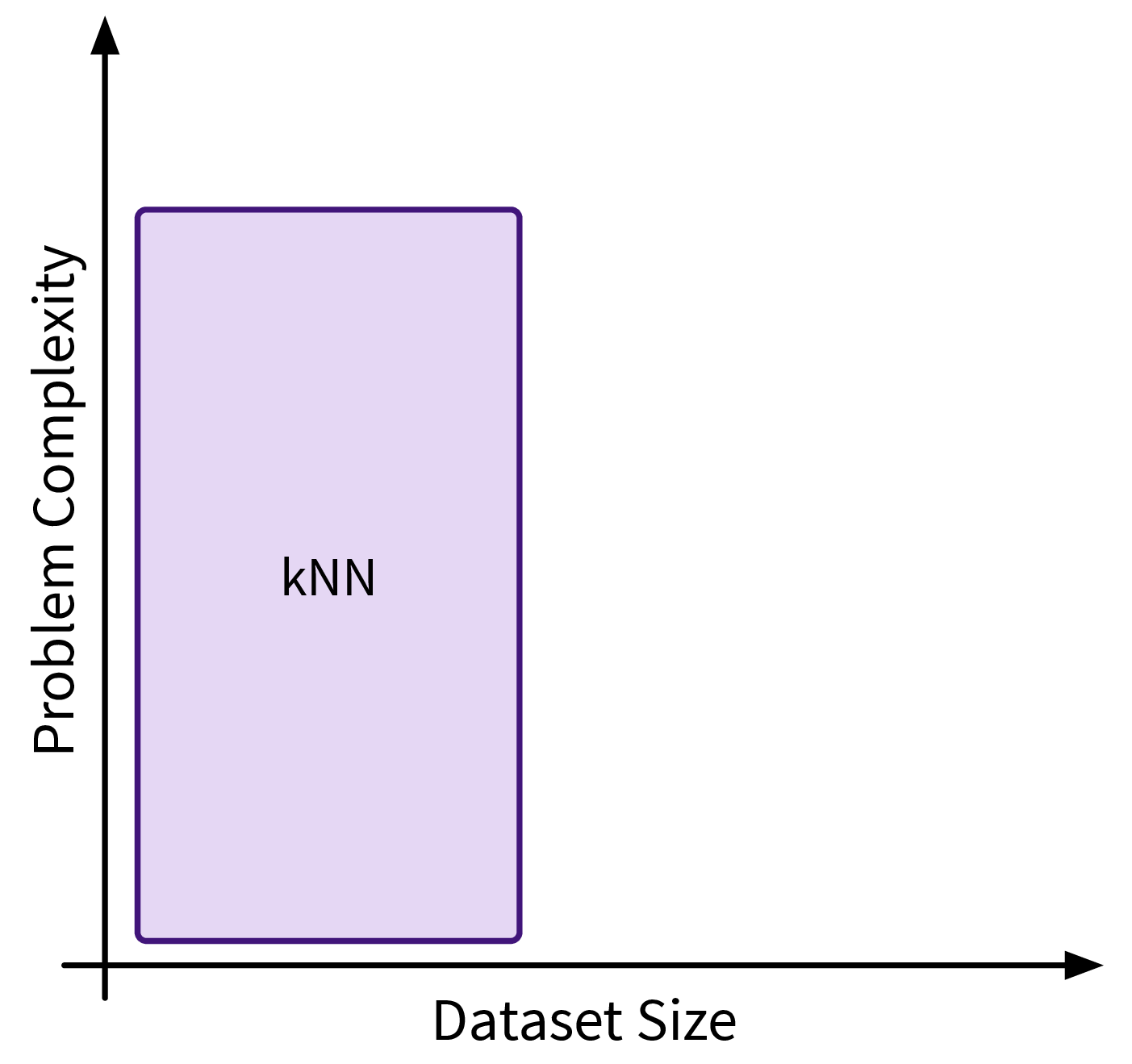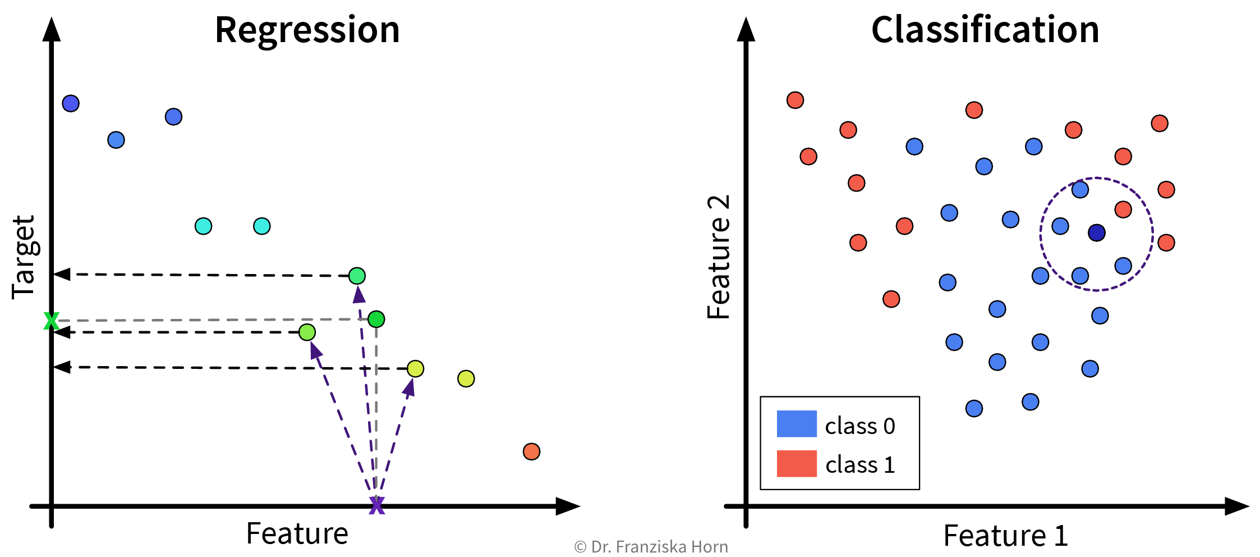### k-Nearest Neighbors (kNN)The first similarity-based model we’ll look at is k-nearest neighbors (kNN), which follows a rather naive and straightforward approach, but nevertheless often achieves a good performance on complex problems.

Main idea

For a new sample, identify the k most similar training data points and predict the average of their target values / their most frequent class:This kind of approach is also called Lazy Learning, since the model doesn’t actually learn any kind of internal parameters, but all the real computation only happens when we make a prediction for a new data point.
(When calling the fit-method on the sklearn model, a search tree is built to efficiently identify the nearest neighbors for a new data point.)

``from sklearn.neighbors import KNeighborsRegressor, KNeighborsClassifier``

Important Parameters:

• `n_neighbors`: How many nearest neighbors to consider when making a prediction.

• `metric`: How to compute the similarity between the samples (default: Euclidean distance).

• `weights`: By setting this to `'distance'` instead of the default `'uniform'`, the labels of the nearest neighbors contribute to the prediction proportionally to their distance to the new data point.

Pros
• Intuitive approach.

Careful
• Results completely depend on the similarity measure.